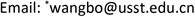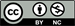Simulation Study on the Influence of Micro Burner Expansion Angle on Hydrogen/Air Premixed Combustion Characteristics<sup> </sup>

Zhuo Wang1, Zhipeng Yu2, Peng Ji2, Bo Wang1*, Menghui Liu1, Chengxi Sun1

1School of Energy and Power Engineering, University of Shanghai for Science and Technology, Shanghai

2Shanghai Marine Diesel Engine Research Institute, ShanghaiReceived: Nov. 20th, 2020; accepted: Feb. 2nd, 2021; published: Feb. 10th, 2021ABSTRACT

Based on the established three-dimensional model of the microburner, the premixed combustion process of hydrogen/air in a 100 mm long microburner with expansion angles of 0˚, 0.36˚ and 0.72˚ was simulated with 10% hydrogen volume fraction. It is found that for square micro-channel burners, with the same burner length, outer wall length and inlet size, the larger the burner expansion angle, the larger the speed limit range. When the inlet velocity is low, the larger the spreading angle is, the lower the flame propagation velocity is, and the higher the maximum combustion temperature is; when the inlet velocity is high and the gas is fully burned, the expansion angle has little effect on the maximum combustion temperature.

Keywords:Micro Burner, Expansion Angle, Inlet Velocity, Maximum Combustion Temperature1. 引言

2. 数值方法与模型2.1. 微型燃烧器的物理模型

Structural parameters of three burner

1113.820800
2123.820800.36
3133.820800.72

1) 预混燃气为不可压缩理想气体，在进入燃烧器时已混合均匀；

2) 忽略气体的辐射作用；

3) 忽略气体的体积力；

4) 忽略燃烧器壁面的催化作用；

5) 忽略浓度梯度产生的粘性热耗散效应和Dufour效应；

6) 忽略入口与出口处壁面带来的环境热损失。

2.2. 微型燃烧器的数学模型

∂ ( ρ u ) ∂ x + ∂ ( ρ v ) ∂ y + ∂ ( ρ w ) ∂ z = 0 (1)

∂ ( ρ u u ) ∂ x + ∂ ( ρ u v ) ∂ y + ∂ ( ρ u v ) ∂ z = − ∂ p ∂ x + ∂ ∂ x ( μ ∂ u ∂ x ) + ∂ ∂ y ( μ ∂ v ∂ y ) + ∂ ∂ z ( μ ∂ w ∂ z ) (2)

∂ ( ρ v u ) ∂ x + ∂ ( ρ v v ) ∂ y + ∂ ( ρ w v ) ∂ z = − ∂ p ∂ y + ∂ ∂ x ( μ ∂ u ∂ x ) + ∂ ∂ y ( μ ∂ v ∂ y ) + ∂ ∂ z ( μ ∂ w ∂ z ) (3)

∂ ∂ x ( k w ∂ T ∂ x ) + ∂ ∂ y ( k w ∂ T ∂ y ) + ∂ ∂ z ( k w ∂ T ∂ z ) = 0 (4)

∂ ( ρ h u ) ∂ x + ∂ ( ρ h v ) ∂ y + ∂ ( ρ h w ) ∂ z = ∂ ∂ x ( k f ∂ T ∂ x ) + ∂ ∂ y ( k f ∂ T ∂ y ) + ∂ ∂ z ( k f ∂ T ∂ z ) + ∑ i ∂ ∂ x ( h i ρ D i . m ∂ Y i ∂ x )       + ∑ i ∂ ∂ y ( h i ρ D i . m ∂ Y i ∂ x ) + ∑ i ∂ ∂ z ( h i ρ D i . m ∂ Y i ∂ x ) + ∑ i h i W i (5)

∂ ( ρ h u ) ∂ x + ∂ ( ρ h v ) ∂ y + ∂ ( ρ h w ) ∂ z = ∑ i ∂ ∂ x ( D i . m ∂ Y i ∂ x ) + ∑ i ∂ ∂ y ( D i . m ∂ Y i ∂ x ) + ∑ i ∂ ∂ z ( D i . m ∂ Y i ∂ x ) + W i (6)

∂ ∂ x ( k w ∂ T ∂ x ) + ∂ ∂ y ( k w ∂ T ∂ y ) + ∂ ∂ z ( k w ∂ T ∂ z ) = 0 (7)

2.3. 反应机理

19 step reaction mechanism between hydrogen and ai

1H 2 + O 2 = 2OH1.70E+130.0047780
2OH + H 2 = H 2 O + H1.17E+091.33626
3H + O 2 = OH + O5.13E+16−0.81616507
4O + H 2 = OH + H1.80E+101.008826
5H + O 2 + M = HO 2 + M a 2.10E+18−1.000.00
6H + O 2 + O 2 = HO 2 + O 26.70E+19−1.420.00
7H + O 2 + N 2 = HO 2 + N 26.70E+19−1.420.00
8OH + HO 2 = H 2 O + O 25.00E+130.001000
9H + HO 2 = 2OH2.5E+140.001900
10O + HO 2 = O 2 + OH4.80E+130.001000
112OH = O + H 2 O6.00E+081.300.00
12H 2 + M = H + H + M b 2.23E+120.5092600
13O 2 + M = O + O + M1.85E+110.5095560
14H + OH + M = H 2 O + M c 7.5E+23−2.600.00
15H + HO 2 = H 2 + O 22.5E+130.00700
16HO 2 + HO 2 = H 2 O 2 + O 22.00E+120.000.00
17H 2 O 2 + M = OH + OH + M1.30E+170.0045500
18H 2 O 2 + H = HO 2 + H 21.60E+120.003800
19H 2 O 2 + OH = H 2 O + HO 21.00E+130.001800

k = A T β exp ( − E K / R T ) (8)

2.4. 边界条件的设置

2.5. 模型验证

1) 将燃烧器的流体通道的中心线上的最高燃烧温度定义为燃烧器的最高燃烧温度。

2) 将燃烧器流体通道的中心线上的OH浓度最高的位置所对应的流体速度定义为燃烧器的火焰传播速度。

3) 氢气与空气的19步反应机理中反应最慢的基元反应是第12步反应，即H2 + M = H + H + Mb，所以将燃烧器流体通道的中心线上，第12步反应最快的位置即为火焰的位置。

3. 结果与分析

3.1. 扩展角对燃烧器中的流速分布的影响

3.2. 扩展角对燃烧器中的温度分布影响

3.3. 扩展角对燃烧器中燃烧产物分布的影响

3.4. 扩展角对燃烧器的火焰位置的影响

4. 结论

1) 扩展角为0.72˚的燃烧器具有更大的速度极限和更低的贫燃极限。其中氢气体积分数为10%时，扩展角为0.72˚的燃烧器的速度极限达到了0.4~15 m/s；扩展角为0.36˚和扩展角为0˚的燃烧器的速度极限为0.4~9 m/s。

2) 扩展角为0˚的燃烧器在入口速度较高时表现出了更低的燃烧温度，更高的火焰传播速度；扩展角为0.72˚的燃烧器在入口速度为11 m/s时火焰中心最靠近出口位置，这对燃烧器的燃烧不利。

3) 三种燃烧器在共有的速度极限范围内不同入口速度下的出口温度相同，不受燃烧器结构的影响。